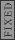GR 8677927796770177 | # Login | Register

GR0177 #91
Problem
 GREPhysics.NET Official Solution Alternate Solutions
This problem is still being typed.
Mechanics}Conservation of Energy

Conservation of energy gives . , and thus the equation becomes

.

Thus, . (Note that the height of the center of mass is the same at both the end and the start, thus the extra bit of the potential energy MgR cancels out. Thanks to the user keflavich for this correction.)

Given , one plugs it into the equation above to get

, as in choice (B).Alternate Solutions
 Ning Bao2008-02-01 08:11:02 Let R, m,h,g=1. Then you just have 1=1/2*8/7+1/2*I*8/7 1=4/7+4/7*I I=3/4.Reply to this commentredmomatt
2011-10-08 15:23:01
Did this by process of elimination (not even looking at the PE versus KE).

(A) can't be true, since the cylinder is not of uniform density.

(D) can't be true, since this would only occur if all of the mass were concentrated in a thin ring at a distance .

(E) can't be true, since the largest moment would be (D).

We are left with (B) and (C). Because a radial mass distribution that varies as should be less than something very close to (D), we are left with choice (B).
 Nezumi2012-02-09 18:27:24 The radial dependence is not given to be linear. I Think (B) or (C) remain until you look at energy.
 Nezumi2012-03-06 12:06:24 I was looking at the problem again. The elimination you did is the right place to start. Once you have it down to (B) and (C), look at the translational energy. since the translation takes up a little more than half of the original energy of the object, less than half is available for the rotational energy. This implies a smaller moment of inertia, hence choice (B)Ning Bao
2008-02-01 08:11:02
Let R, m,h,g=1. Then you just have

1=1/2*8/7+1/2*I*8/7
1=4/7+4/7*I
I=3/4.
 smokwzbroiplytowej2008-10-21 11:27:20 Nice - sure helps save time! Caveat: you really can't set and simultaneously, since they are both measures of length. Here it works rather nicely, because H cancels out as you could tell from the answers.neutrino
2007-10-31 03:28:11
You can make the calculation more easy by noting that the rotational inertia will look like

,

with some constant. Now, you just have to solve:

This might save you some time. Because, 1.7 min..., man, that is not much time.
 tommy222222019-10-22 14:57:59 BRILLIANT!!! I think this is the best and the most make sense alternative solution under this pose.Richard
2007-10-30 18:38:31
A potentially useless note:

The solution given in (E) is found by NOT accounting for the translational kinetic energy.Andresito
2006-03-05 00:00:42
Yosun, there is a typo on your solution. When you express I, the term MV^2 has a factor of 2 implicitly.

Recall the conservation of energy equation, multiply both terms by 2 and the "only" term that has now a factor of 2 is MgH.

The correct expression should have I=(2 MgH - MV^2) (r/v)^2

Yosun, thank you very much for working out the problems. If you are a girl I send you a big kiss :). I obtained the pdf from the yahoo-group. It also has the typo.keflavich
2005-11-10 22:48:10
You don't need to consider as part of the gravitational potential energy. When the cylinder reaches the bottom of the plane, its center of mass will remain at a distance R above the ground.
 yosun2005-11-10 22:54:19 keflavich: you're right that the center of mass stays at a height of R above the ground at both points. thanks for the correction.LaTeX syntax supported through dollar sign wrappers $, ex.,$\alpha^2_0$produces . type this... to get...$\int_0^\infty\partial\Rightarrow\ddot{x},\dot{x}\sqrt{z}\langle my \rangle\left( abacadabra \right)_{me}\vec{E}\frac{a}{b}\$